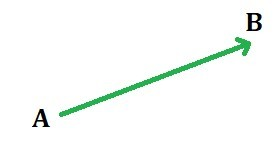Related Articles
Vector Projection using Python
• Last Updated : 29 Nov, 2019

A vector is a geometric object which has both magnitude (i.e. length) and direction. A vector is generally represented by a line segment with a certain direction connecting the initial point A and the terminal point B as shown in the figure below and is denoted by## Projection of a Vector on another vector

The projection of a vectoronto another vectoris given asComputing vector projection onto another vector in Python:

 # import numpy to perform operations on vector  import numpy as np     u = np.array([1, 2, 3])   # vector u  v = np.array([5, 6, 2])   # vector v:     # Task: Project vector u on vector v     # finding norm of the vector v  v_norm = np.sqrt(sum(v**2))         # Apply the formula as mentioned above  # for projecting a vector onto another vector  # find dot product using np.dot()  proj_of_u_on_v = (np.dot(u, v)/v_norm**2)*v     print("Projection of Vector u on Vector v is: ", proj_of_u_on_v)

Output:

Projection of Vector u on Vector v is:  [1.76923077 2.12307692 0.70769231]

One liner code for projecting a vector onto another vector:

 (np.dot(u, v)/np.dot(v, v))*v

## Projection of a Vector onto a Plane

The projection of a vectoronto a plane is calculated by subtracting the component ofwhich is orthogonal to the plane from.where,is the plane normal vector.

Computing vector projection onto a Plane in Python:

 # import numpy to perform operations on vector  import numpy as np     # vector u   u = np.array([2, 5, 8])            # vector n: n is orthogonal vector to Plane P  n = np.array([1, 1, 7])             # Task: Project vector u on Plane P     # finding norm of the vector n   n_norm = np.sqrt(sum(n**2))          # Apply the formula as mentioned above  # for projecting a vector onto the orthogonal vector n  # find dot product using np.dot()  proj_of_u_on_n = (np.dot(u, n)/n_norm**2)*n     # subtract proj_of_u_on_n from u:   # this is the projection of u on Plane P  print("Projection of Vector u on Plane P is: ", u - proj_of_u_on_n)

Output:

Projection of Vector u on Plane P is:  [ 0.76470588  3.76470588 -0.64705882]My Personal Notes arrow_drop_up
Recommended Articles
Page :